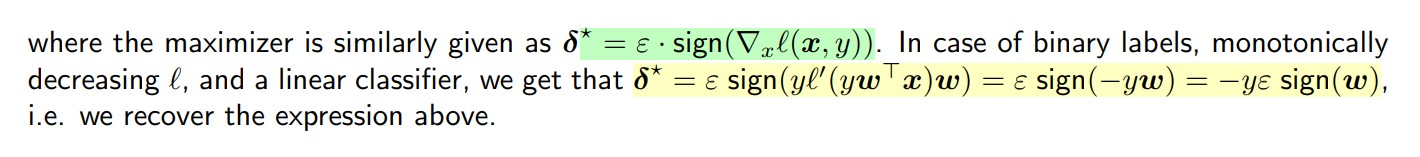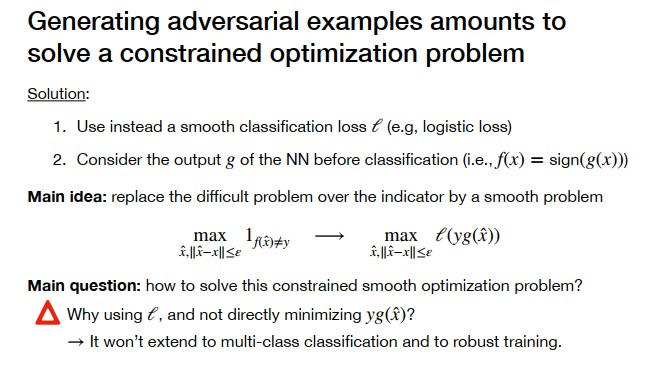### Lab 10 Problem 1 point 3 : theory FSGM and gradient

In lab 10 (problem 1, point 3) I don't understand how the solution of of FSGM problem was derived, more precisely how do we go from the formula highlighted in green to the formula highlighted in yellow.In slide 12 on lecture 9c we get the formula in green but I can't figure out which loss we are taking the gradient of here, won't we need subgradients to calculate the hinge loss gradient ?

Top comment

I agree with El Mahdi's comment: basically, taking the $$sign$$ of a negative $$l' < 0$$ gives you -1.

However, I think the original question was also about the corner case of $$l' = 0$$ as could happen in the hinge loss when the margin $$z > 1$$. I think this case is indeed problematic as then the whole gradient $$\nabla_x l(x, y)$$ will be equal to zero, thus making FGSM meaningless (as we won't move at all due to the zero gradient). Thus, the FGSM part actually requires $$l'$$ to be strictly negative to rule out the corner cases such as with the hinge loss. We should update the solution of this exercise accordingly.

Update based on the other comment: smoothness is not a necessary requirement per se, we just need the derivative $$l'$$ to be strictly negative and it's fine to have some non-differentiable points if subgradient exists in them.

Supposing it was hinge loss, to me a subgradient for it would be l'(z) = 0 for z>1 and l'(z) =-1 for z<=1 so I don't see how l' it is reduced to -1 at the before last step

I will assume you have no problem arriving at the green equation.
Now as it is clearly stated, we consider the special case of a binary classification problem with a decreasing loss $$l$$. Remember that in this case : $$l(x,y)= l(yw^\top x)$$ so that $$\nabla_x l(x,y)= y l'(yw^\top x) w$$ and $$l'\leq 0$$ because $$l$$ is decreasing.

It should be clear by now why things simplify into the yellowish equation.

Thank you! It's clearer now but I'm not sure of one thig :
The property you gave, comes from the fact that we want to use a smooth loss or is it a generality that we should know? I couldn't find it outside of lecture 9, I mean "binary classification problem with a decreasing loss. Remember that in this case $$l(x, y)=l\left(y w^{\top} x\right)$$"

where I think it was mentioned in lecture 9 : ( slide 9)I agree with El Mahdi's comment: basically, taking the $$sign$$ of a negative $$l' < 0$$ gives you -1.
However, I think the original question was also about the corner case of $$l' = 0$$ as could happen in the hinge loss when the margin $$z > 1$$. I think this case is indeed problematic as then the whole gradient $$\nabla_x l(x, y)$$ will be equal to zero, thus making FGSM meaningless (as we won't move at all due to the zero gradient). Thus, the FGSM part actually requires $$l'$$ to be strictly negative to rule out the corner cases such as with the hinge loss. We should update the solution of this exercise accordingly.
Update based on the other comment: smoothness is not a necessary requirement per se, we just need the derivative $$l'$$ to be strictly negative and it's fine to have some non-differentiable points if subgradient exists in them.